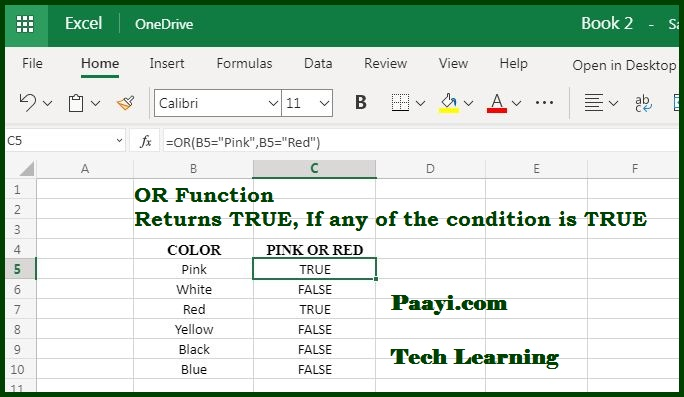# Learn How to Use Microsoft Excel OR Function

Written by | 0 Comments | 778 Views

In this article, you will learn how to use the Microsoft Excel OR function and its prime function in Microsoft Excel. You will also get to know the Microsoft Excel OR function return value and syntax with the help of some examples.

## Microsoft Excel OR Function

The Microsoft Excel OR function allows you to test multiple conditions. The OR function is the logical function, and with it, you can try the numerous conditions at the same time. Suppose you want to test either "A" or "B" you can use =OR(A1="A", A1="B"). So, it is a very simple function and can be used inside the IF function so that you can avoid extra nested IF's. You can always combine the OR function can also be combined with the AND function.

### OR Function Return Value

The OR function returns TRUE if any one of the arguments evaluates TRUE and FALSE if both of them are FALSE.

### OR Function Syntax

=OR(logical1,[logical2],...)

Where:

• logical1: This is the first condition or the logical value you want to evaluate.
• logical2: This is the second condition or logical value you want to consider (optional).

## How to Use Microsoft Excel OR Function?You can use the OR function to test up to 255 multiple conditions. Each logical condition must return either TRUE or FALSE, or it can be arrays or references containing the logical values. The OR function examine all the values, and return TRUE if any one of the value is TRUE, in the same way, if not any value evaluates to be TRUE, the OR function will return FALSE.